# Depth-First Search Algorithms

searching from root to leaves

The depth-first search algorithm is a handy algorithm highly applicable to a wide variety of computer science problems. This algorithm involves searching through a sequence of nodes in a tree or data structure. However, unlike a breadth-first search, a depth-first search follows a different pattern. In the depth-first search pattern, the algorithm goes ‘deep’ instead of ‘wide.’ In other words, it searches for a path from the root node to the lowest leaf before moving onto the next possible path.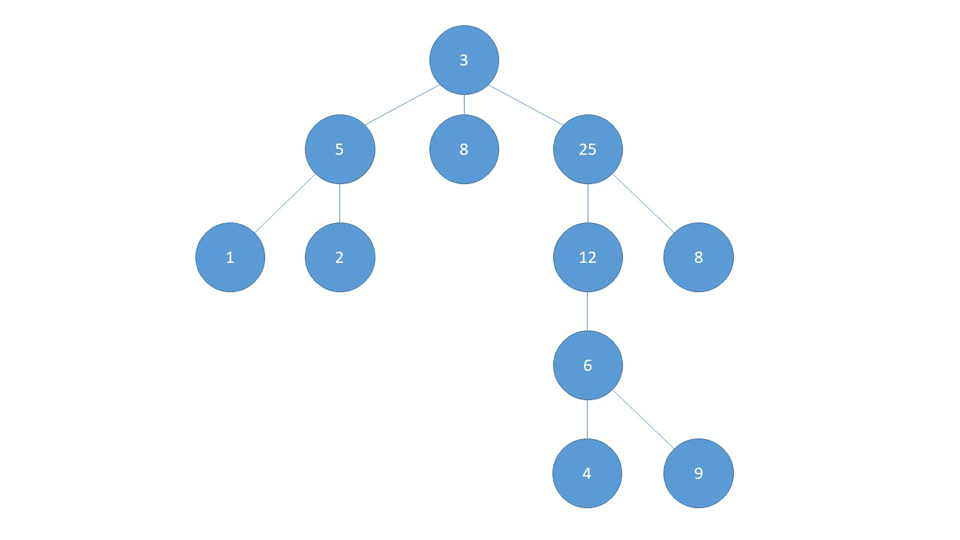Depth-First Search Graphic

The animation above shows the flow of depth-first search. The algorithm finds all paths from the root node to the lowest leaves by going as deep as possible and then backtracking to find the next lowest leaf. If not exited, both depth-first search and breadth-first search algorithms visit every node, just in a different order. Depth-first search algorithms can solve many of the same problems as breadth-first search, although depth-first is well suited to find paths in a graph or maze, scheduling optimization, and topological sorting.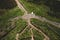Photo by Tara Scahill on Unsplash

The broad steps of the algorithm for the depth-first search is as follows:

A. DFS Recursive Implementation
1. procedure DFS(g=graph,v=node being visited)
2. Base Case=if node is a leaf with no children mark as visited and return
3. LOOP through all adjacent nodes
i.For each node, mark as visited and recursively call procedure DFS on a new node

B.DFS Iterative Implementation
1. procedure DFS(g=graph,v=node being visited)
2. Create a Stack to store nodes.
3. mark node v as visited and push onto the stack.
4. LOOP while the stack is not empty.
i. if a node is a leaf, or its children are marked visited: pop the node off the top of the stack
ii. else: push all of its children onto the stack in the proper order
5.END LOOP

Let’s talk about what this means when solving a problem in more detail by looking at an example we explored in the previous ‘Bread-First Search Algorithm’ article.

Problem: Given a tree of nodes containing integers. Find the total number of even integers in the node.

Input: root node of a tree of integers

Output: number of even numbers in all of the tree’s nodes.

Let’s take the tree below as input: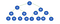Tree with integers stored in nodes

Looking at the tree above, we can see that this tree has four levels with 15 nodes. The problem asks us to visit each of these nodes traversing depth-first. As the algorithm traverses the tree, it should count the number of even numbers visited and return that number at the end. However, before we delve deeper into the algorithm, we need to get into more detail about the data structures we must use.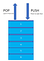Stack Data Structure

To understand how the depth-first search process works, we must grasp the stack data structure. With the iterative approach, we must create a stack data structure explicitly. With the recursive method, the stack data structure is created implicitly by calling recursive functions. Either way, examining the stack helps us understand how the depth-first search processes the nodes in order.

Stacks follow the Last In First Out(LIFO) principle. The stack adds elements to itself by pushing those elements on top of the stack. At the same time, the stack pops datum by removing them from the top of the stack. Unlike a Queue that removes and adds elements at different ends, a stack adds and removes elements at the same end.

The order by which the algorithm processes nodes in the stack determines the order of traversal. The three most common ways of traversing nodes in a depth-first search are pre-order transversal, in-order transversal, and post-order transversal.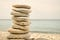Photo by Iva Rajović on Unsplash

In pre-order traversal, the function marks nodes of the tree as visited in the following order: [Root Node] — [Left Node] — [Right Node]. In other words, starting at a specific node, the algorithm checks that node first. Next, the method checks all nodes to the left of that node in the tree. Finally, the program checks all nodes to the right.

5 -> 3 -> 99 -> 9 -> 10 -> 88 -> 78 -> 98 -> 66 -> 80 -> 4 -> 8 -> 55 -> 88 -> 3

Looking at the number of nodes and the ordered numbers above it, we see that at each level, the algorithm visits the middle node first, followed by all of the nodes to the left and finally all nodes to the right.

Root Node —  -> Left Nodes — [3 -> 99 -> 9 -> 10 -> 88 -> 78 -> 98] -> Right Nodes — [66 -> 80 -> 4 -> 8 -> 55 -> 88 -> 3]

In-order transversal, the method visits the nodes in the following basic order: [Left Node] — [Root Node] — [Right Node]. In other words, starting at a specific node, it visits all of the nodes to the left, followed by the node itself and finally all of the nodes to the right.

9 -> 99 -> 10 -> 3 -> 78 -> 88 -> 98-> 4->80->8->66->88->55->3

Looking at the number of nodes and the ordered numbers above it, we see that the nodes to the left of the root are visited at each level, followed by the node and finally all nodes to the right.

Left Nodes — [9 -> 99 -> 10 -> 3 -> 78 -> 88 -> 98]->Root Node->Right Nodes — [4->80->8->66->88->55->3]

Post-order transversal, the algorithm visits the nodes in the following order: [Left Node] — [Right Node] — [Root Node]. In other words, starting at a specific node, all of the nodes to its left are checked, then nodes to the right, followed by the root node.

9 -> 10 -> 99 -> 78 -> 98 -> 88 -> 3 -> 4 -> 8 -> 80 -> 88 -> 3 -> 55 -> 66 -> 5

Looking at the number of nodes and the ordered numbers above it, we see that the nodes to the left of the root are visited at each level, followed by all the nodes to the right and then the root node itself.

Left Nodes-[9 -> 10 -> 99 -> 78 -> 98 -> 88 -> 3 -> 4] -> Right Nodes-[8 -> 80 -> 88 -> 3 -> 55 -> 66 ]->Root Node-

For this problem, we will be using pre-order transversal. Setting this up properly with our stack requires the pushes and pops to be conducted in the right order. The underlying logic is as follows:

1. Starting at the root node, mark the node as visited and push onto the stack.
2. If the left node exists and is not visited, mark it as visited and push it onto the stack. If this node has no children,i.e., is a leaf, then pop it off the stack.
3. If the left node either doesn’t exist or has been visited, visit the right node.
4. If both child nodes are marked visited, pop the node off the stack.
5. Continue until the stack is empty, and all nodes are marked visited.

Let’s examine how this would work with our example.

Keeping in mind our rules above, the pre-order transversal for the tree above will move as follows.

Our output will be ‘9’ with the numbers being [10,88,78,98, 66,80,4,8,88] for our specific example of finding all of the even numbers. As stated before, we could implement this algorithm either iteratively or recursively. With the iterative solution, the code would require a stack data structure and an algorithm that ensures the pushes and pops' proper timing. On the other hand, a recursive implementation is, in many ways, much more straightforward with the stack structure implicit through the recursive function calls and returns.

Recursive Implementation

1. Base Case: if both children have been visited or don’t exist, return
2. Else: mark node as visited
3. recursive call to DFS(node-> right)
4. recursive call to DFS(node -> left)

Calculating the time complexity of DFS is fairly straightforward. Similar to BFS, DFS visits each node once. In the worst case, it visits all of the tree’s nodes. Therefore, the algorithm time will be proportional to the number of nodes in the tree or O(n).

Calculating the space complexity is slightly more involved. The size of the stack will determine the memory occupied by the algorithm. The tree's height will limit the size of the stack at any given time since the algorithm moves to the deepest leaf. In other words, the space complexity is O(h), where H is the height of the tree.

Can we find a relationship between h and n? Looking at the table below, we can find an approximate pattern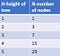Relationship of tree height and nodes

The numbers above follow a pattern where the number of nodes is approximately 2 to the power of h(2^h). Consequently, the space taken up in memory will be a logarithm of the number of nodes in base 2. Thus, the space complexity is O(log n).

1. Depth-First-Search works by searching a tree data structure deep instead of broad by going as deep as possible before returning.
2. A DFS algorithm can be implemented recursively or iteratively. An iterative implementation usually requires a loop with a stack data structure. A recursive implementation does not require a stack but relies on the correct ordering of function calls and returns.
3. The three most common traversal orders for DFS are pre-order traversal, post-order traversal, and in-order traversal.
4. The time and space complexity for DFS is O(n) and O(log n), respectively.

## The Startup

Get smarter at building your thing. Join The Startup’s +785K followers.

### By The Startup

Get smarter at building your thing. Subscribe to receive The Startup's top 10 most read stories — delivered straight into your inbox, once a week. Take a look.

By signing up, you will create a Medium account if you don’t already have one. Review our Privacy Policy for more information about our privacy practices.

Check your inbox
Medium sent you an email at to complete your subscription.

Written by

## Adam Shaffer

A full-stack software developer who likes writing about tech.

## The Startup

Get smarter at building your thing. Follow to join The Startup’s +8 million monthly readers & +785K followers.

Written by

## Adam Shaffer

A full-stack software developer who likes writing about tech.## The Startup

Get smarter at building your thing. Follow to join The Startup’s +8 million monthly readers & +785K followers.

Medium is an open platform where 170 million readers come to find insightful and dynamic thinking. Here, expert and undiscovered voices alike dive into the heart of any topic and bring new ideas to the surface. Learn more

Follow the writers, publications, and topics that matter to you, and you’ll see them on your homepage and in your inbox. Explore

If you have a story to tell, knowledge to share, or a perspective to offer — welcome home. It’s easy and free to post your thinking on any topic. Write on Medium